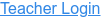PhET Interactive Simulations
University of Colorado Boulder

### Topics

• Factors
• Products
• Area Model
• Multiplication
• Partial Products

### Description

Build rectangles of various sizes and relate multiplication to area. Partition a rectangle into two areas to discover the distributive property.

### Sample Learning Goals

• Recognize that area represents the product of two numbers.
• Develop and justify a strategy that uses the area model to simplify a multiplication problem.
• Represent a multiplication problem as the proportional area of a rectangle.
• Looks for patterns in the total area calculation.
Version 1.0.0